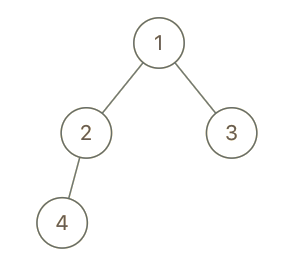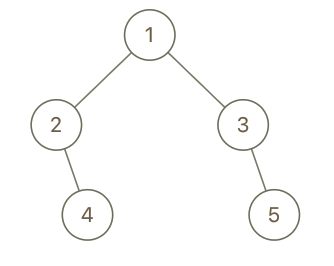# 993. Cousins in Binary Tree

## 993. Cousins in Binary Tree

Given the `root` of a binary tree with unique values and the values of two different nodes of the tree `x` and `y`, return `true` if the nodes corresponding to the values `x` and `y` in the tree are cousins, or `false` otherwise.

Two nodes of a binary tree are cousins if they have the same depth with different parents.

Note that in a binary tree, the root node is at the depth `0`, and children of each depth `k` node are at the depth `k + 1`.

Example 1:```Input: root = [1,2,3,4], x = 4, y = 3
Output: false
```

Example 2:```Input: root = [1,2,3,null,4,null,5], x = 5, y = 4
Output: true
```

Example 3:```Input: root = [1,2,3,null,4], x = 2, y = 3
Output: false
```

Constraints:

• The number of nodes in the tree is in the range `[2, 100]`.
• `1 <= Node.val <= 100`
• Each node has a unique value.
• `x != y`
• `x` and `y` are exist in the tree.

### The solution to the above question

/**
* Definition for a binary tree node.
* struct TreeNode {
*     int val;
*     TreeNode *left;
*     TreeNode *right;
*     TreeNode() : val(0), left(nullptr), right(nullptr) {}
*     TreeNode(int x) : val(x), left(nullptr), right(nullptr) {}
*     TreeNode(int x, TreeNode *left, TreeNode *right) : val(x), left(left), right(right) {}
* };
*/
class Solution {
public:

bool isCousins(TreeNode* rootint xint y) {
queue<TreeNode*>q;

TreeNode* xp;
int dpx=1;
int dpy=1;
TreeNode* yp;
int depth=0;
q.push(root);
q.push(NULL);
if(root->val==x){
xp=root;
dpx=depth;
}
else if(root->val==y){
yp=root;
dpy=depth;
}
while(!q.empty()){
TreeNode* f=q.front();
if(f!=NULL){
if(f->left){
q.push(f->left);
if(f->left->val==x){
xp=f;
dpx=depth;
}
else if(f->left->val==y){
yp=f;
dpy=depth;
}
}
if(f->right){
q.push(f->right);
if(f->right->val==x){
xp=f;
dpx=depth;
}
else if(f->right->val==y){
yp=f;
dpy=depth;
}
}
q.pop();

}
else{

depth++;
q.pop();
if(!q.empty()){
q.push(NULL);
}
}

}
if(xp!=yp && dpy==dpx){
return true;
}
return false;

}
};